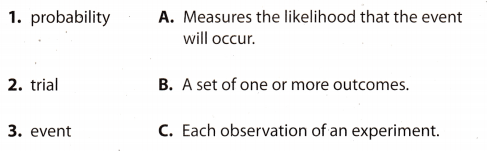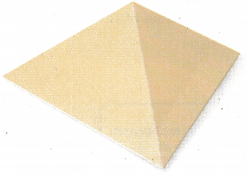# Texas Go Math Grade 7 Module 5 Answer Key Experimental Probability

Refer to our Texas Go Math Grade 7 Answer Key Pdf to score good marks in the exams. Test yourself by practicing the problems from Texas Go Math Grade 7 Module 5 Answer Key Experimental Probability.

## Texas Go Math Grade 7 Module 5 Answer Key Experimental Probability

Write each fraction in simplest form.

Question 1.
$$\frac{6}{10}$$ ____________
Find the greatest common factor of the numerator and denominator.
List factors of the numerator and denominator.
6 : 1, 2, 3, 6
10 : 1, 2, 5, 10
The greatest common factor is 2.
Divide the numerator and denominator by the greatest common factor.
$$\frac{6 \div 2}{10 \div 2}$$ = $$\frac{3}{5}$$

$$\frac{9}{15}$$ ____________
Find the greatest common factor of the numerator and denominator.
List factors of the numerator and denominator.
9 : 1, 3, 9
15 : 1, 3, 5, 15
The greatest common factor is 3.
Divide the numerator and denominator by the greatest common factor.
$$\frac{9 \div 3}{15 \div 34}$$ = $$\frac{3}{5}$$

Question 3.
$$\frac{16}{24}$$ ____________
Find the greatest common factor of the numerator and denominator.
List factors of the numerator and denominator.
16 : 1, 2, 4, 8, 16
24 : 1, 2, 3, 4, 6, 8, 12, 24
The greatest common factor is 8.
Divide the numerator and denominator by the greatest common factor.
$$\frac{16 \div 8}{24 \div 8}$$ = $$\frac{2}{3}$$

Question 4.
$$\frac{9}{36}$$ ____________
Find the greatest common factor of the numerator and denominator.
List factors of the numerator and denominator.
9 : 1, 3, 9
36 : 1, 2, 3, 4, 6, 9, 12, 18, 36
The greatest common factor is 9.
Divide the numerator and denominator by the greatest common factor.
$$\frac{9 \div 9}{36 \div 9}$$ = $$\frac{1}{4}$$

Question 5.
$$\frac{45}{54}$$ ____________
Find the greatest common factor of the numerator and denominator.
List factors of the numerator and denominator.
45 : 1, 3, 5, 9, 15, 45
54 : 1, 2, 3, 6, 9, 18, 27, 54
The greatest common factor is 9.
Divide the numerator and denominator by the greatest common factor.
$$\frac{45 \div 9}{54 \div 9}$$ = $$\frac{5}{6}$$

Go Math Grade 7 Module 5 Topic A Quiz Answer Key Question 6.
$$\frac{30}{42}$$ ____________
Find the greatest common factor of the numerator and denominator.
List factors of the numerator and denominator.
30 : 1, 2, 3, 5, 6, 10, 15, 30
42 : 1, 2, 3, 6, 7, 14, 21, 42
The greatest common factor is 6.
Divide the numerator and denominator by the greatest common factor.
$$\frac{30 \div 6}{42 \div 6}$$ = $$\frac{5}{7}$$

Question 7.
$$\frac{36}{60}$$ ____________
Find the greatest common factor of the numerator and denominator.
List factors of the numerator and denominator.
36 : 1, 2, 3, 4, 6, 9, 12, 18, 36
60 : 1, 2, 3, 4, 5, 6, 10, 12, 15, 20, 30, 60
The greatest common factor is 12.
Divide the numerator and denominator by the greatest common factor.
$$\frac{36 \div 12}{60 \div 12}$$ = $$\frac{3}{5}$$

Question 8.
$$\frac{14}{42}$$ ____________
Find the greatest common factor of the numerator and denominator.
List factors of the numerator and denominator.
14 : 1, 2, 7, 14
42 : 1, 2, 3, 6, 7, 14, 21, 42
The greatest common factor is 14.
Divide the numerator and denominator by the greatest common factor.
$$\frac{14 \div 14}{42 \div 14}$$ = $$\frac{1}{3}$$

Write each fraction as a decimal.

Question 9.
$$\frac{3}{4}$$ ____________
Divide 3 by 4. Write a decimal point and insert extra zeros in the dividend.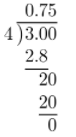The result is 0.75.

$$\frac{7}{8}$$ ____________
Divide 7 by 8. Write a decimal point and insert extra zeros in the dividend.The result is 0.875.

Question 11.
$$\frac{3}{20}$$ ____________
Divide 3 by 20. Write a decimal point and insert extra zeros in the dividend.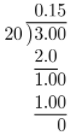The result is 0.15.

Question 12.
$$\frac{19}{50}$$ ____________
Divide 19 by 50. Write a decimal point and insert extra zeros in the dividend.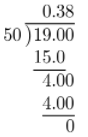The result is 0.38.

Write each percent as a decimal.

Question 13.
67% ______
Write the 67% as the sum of the 1 whole and the percent remainder.
= 100% – 33% Write the percents as a fractions.
= $$\frac{100}{100}$$ – $$\frac{33}{100}$$ Divide.
= 1 – 0.33 Subtract
= 0.67

Question 14.
31% ______
Write the 31% as the sum of the 1 whole and the percent remainder.
= 100% – 69% Write the percents as a fraction.
= $$\frac{100}{100}$$ – $$\frac{69}{100}$$ Divide.
= 1 – 0.69 Subtract
= 0.31

Question 15.
7% ____________
Write the 7% as the sum of the 1 whole and the percent remainder.
= 100% – 93% Write the percents as a fraction.
= $$\frac{100}{100}$$ – $$\frac{93}{100}$$ Divide.
= 1 – 0.93 Subtract
= 0.7

Question 16.
54% ____________
Write the 54% as the sum of the 1 whole and the percent remainder.
= 100% – 46% Write the percent as a fraction.
= $$\frac{100}{100}$$ – $$\frac{46}{100}$$ Divide.
= 1 – 0.46 Subtract
= 0.54

Write each decimal as a percent.

Question 17.
0.13 ____________
Multiply 0.13 by 1. Any number will be the same when multiplied by 1.
0.13 ∙ 1 = 0.13 ∙ $$\frac{100}{100}$$ Write 1 as a fraction with the denominator 100.
= $$\frac{13}{100}$$ Multiply Write fraction as a percent
= 13%

Question 18.
0.55 ____________
Multiply 0.55 by 1. Any number will be the same when multiplied by 1.
0.55 ∙ 1 = 0.55 ∙ $$\frac{100}{100}$$ Write 1 as a fraction with the denominator 100.
= $$\frac{55}{100}$$ Multiply Write fraction as a percent
= 55%

Question 19.
0.08 ____________
Multiply 0.08 by 1. Any number will be the same when multiplied by 1.
0.08 ∙ 1 = 0.08 ∙ $$\frac{100}{100}$$ Write 1 as a fraction with the denominator 100.
= $$\frac{8}{100}$$ Multiply Write fraction as a percent
= 8%

1.16 ____________
Multiply 1.16 by 1. Any number will be the same when multiplied by 1.
1.16 ∙ 1 = 1.16 ∙ $$\frac{100}{100}$$ Write 1 as a fraction with the denominator 100.
= $$\frac{116}{100}$$ Multiply Write fraction as a percent
= 116%

Visualize Vocabulary

Use the ✓ words to complete the graphic. You can put more than one word in each box.Understand Vocabulary

Match the term on the left to the definition on the right.